# What is the Arithmetic paper in Year 6 SATs?

The Arithmetic paper in the Year 6 SATs, is one of three separate papers that make up the Year 6 Maths SATs. Paper 1 is an Arithmetic paper whilst Paper 2 & 3 are Reasoning papers.  The arithmetic paper mainly tests skills in calculations, fractions, decimals & percentages. The image  below shows the share of marks coming from different maths areas in the last SATs Paper 1.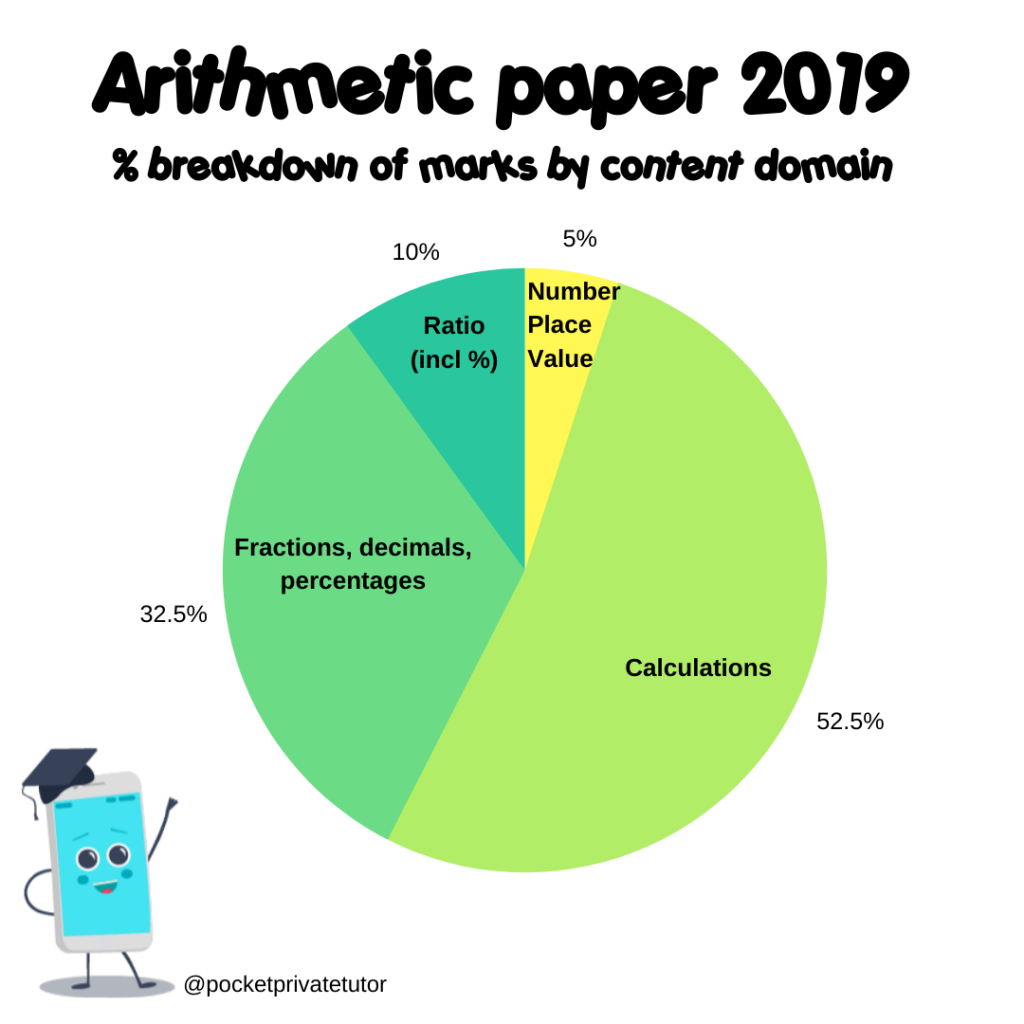## When is the Key Stage 2 SATs Maths Arithmetic paper?

This school year (2021-2022) the arithmetic paper will take place mid Wednesday May 11th 2022 and will take 30 minutes to complete.

## How different is the Arithmetic paper to the reasoning papers?

The format of the questions are very different in the arithmetic and reasoning papers. The arithmetic questions are more straightforward. There are no word problems. The children are simply given a number sentence to solve. Many children score higher on the arithmetic paper because it is a bit clearer to understand what skills they need to apply.

Take a look at Q15 from the Arithmetic Paper and then compare it to Q10 from Reasoning Paper 2 in 2019.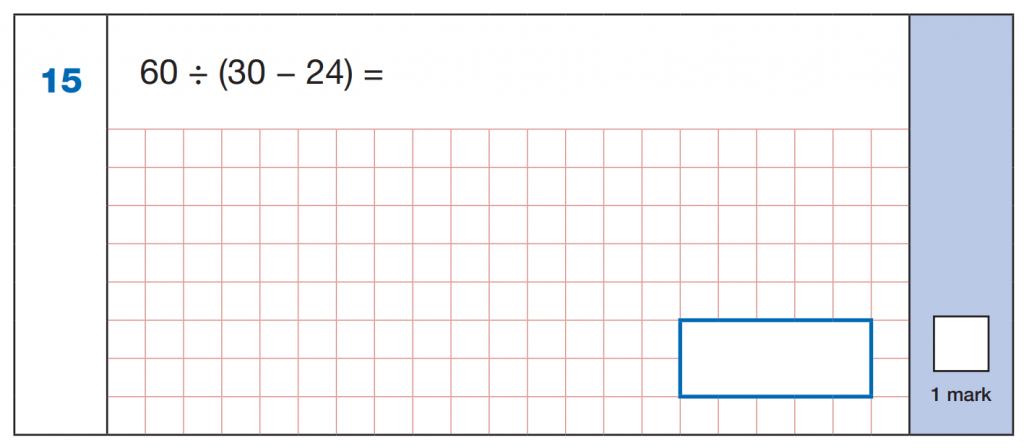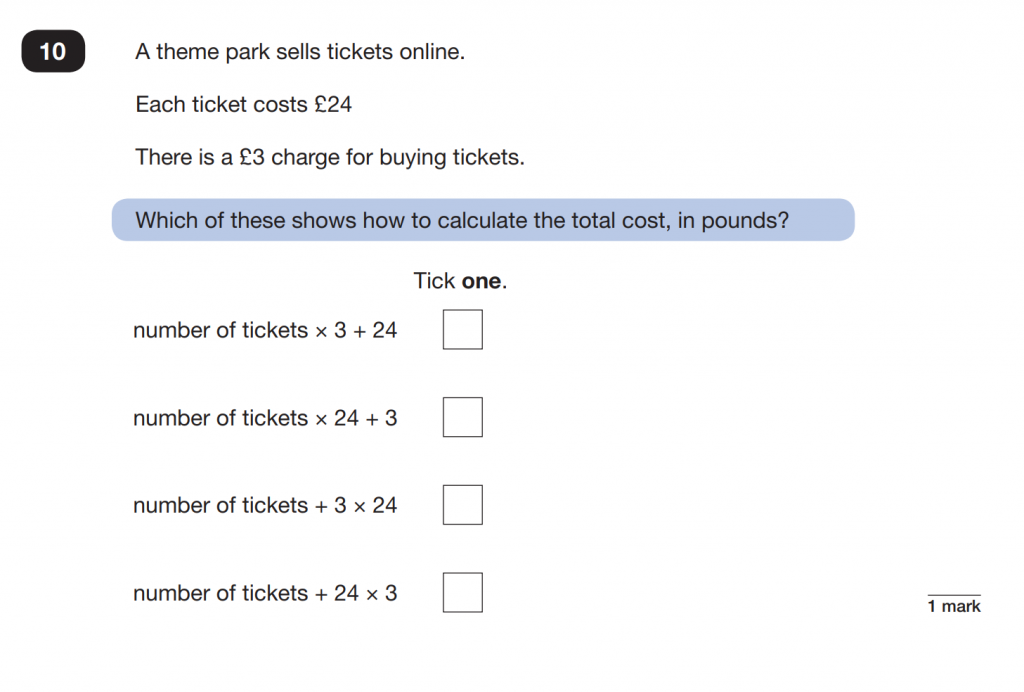Both questions assess knowledge of “order of operations” You can clearly see in the arithmetic paper, it is more obvious what the children need to do. The question in Paper 2 combines “order of operations” with “simple formulae expressed in words”. Questions in the reasoning papers often combine at least two different maths skills. Children will have to do a bit more work in first understanding the problem and then choosing what math skills they need to use!

Now let’s compare Q25 from Paper 1 with Q20 from Paper 3 in 2019 and again you will see it is really clear in Paper 1 what math skill is being tested.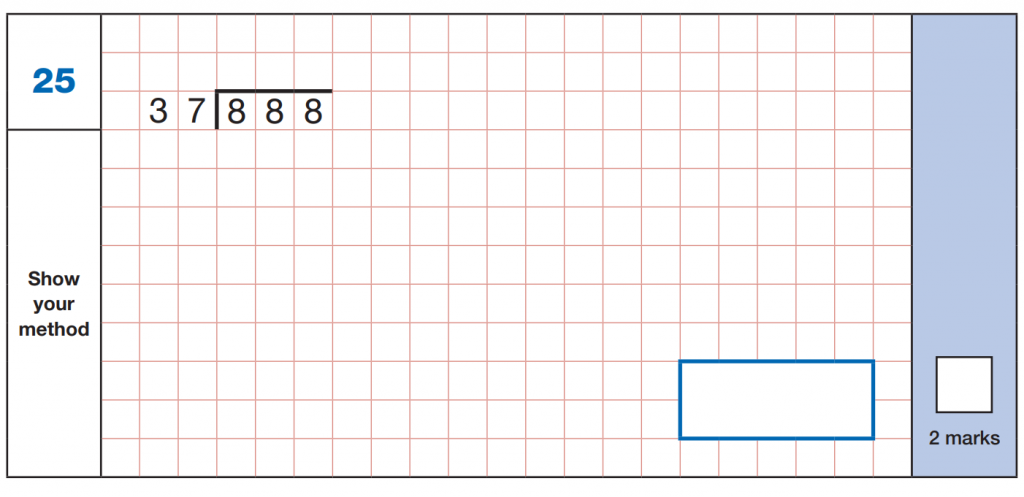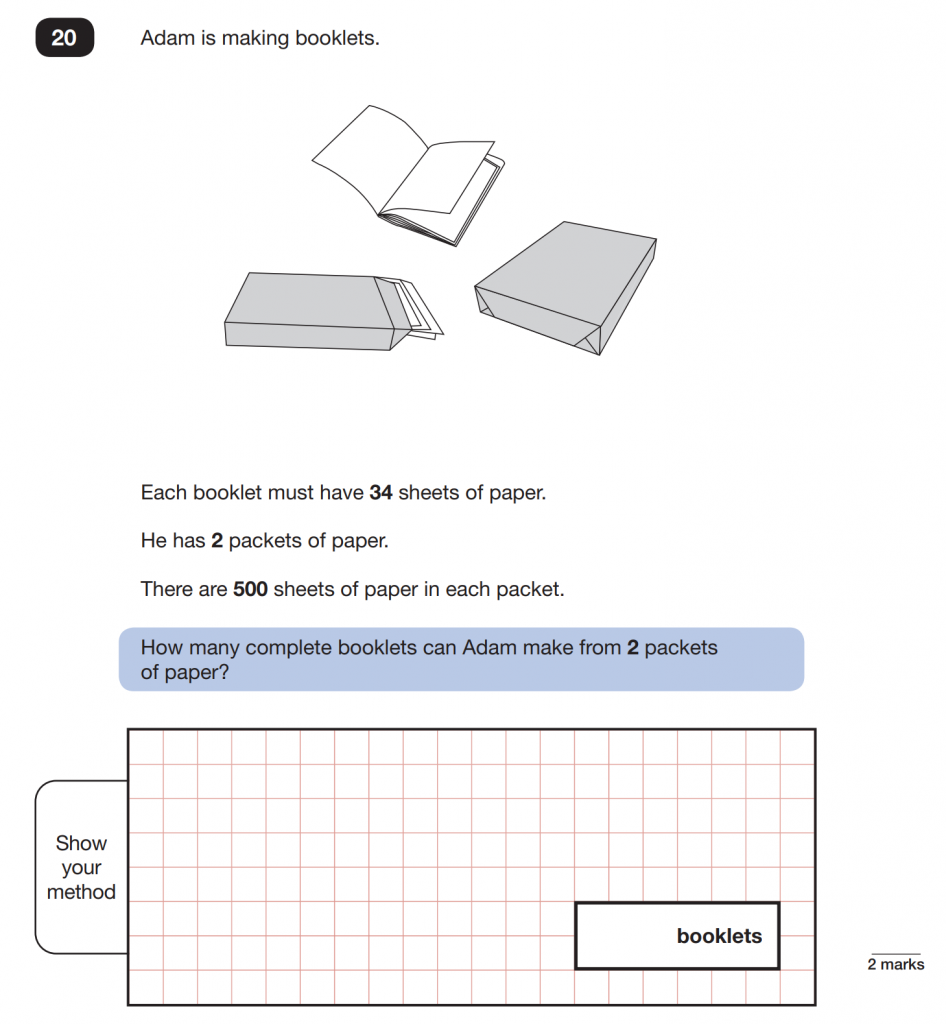In Paper 1, children can also see which numbers they need to divide and with the method set out for them, they have an extra memory jog. In Paper 3, the skill of long division is needed but only after multiplying the packs of paper by 2 to find the total amount of sheets. It is not uncommon to see answers where children have completed accurate calculations BUT they didn’t complete the correct calculation because they misunderstood the question. They could have calculated 500 ÷ 34 or 34 x 2. The answer also required children to understand what to do with their remainder. They would have got the answer 29 with a remainder of 14 or as decimal number. They need to understand that as the question calls for complete booklets, the answer in this context would be 29. Another word problem with the same calculations but a different context could have altered the answer to 30.

## Why is it important to revise for the Key Stage 2 SATs Maths Arithmetic paper?

There are 40 marks available from this paper alone, making up a significant 36% of the total mark.

Arithmetic skills are the building blocks of maths.

Practising these skills will help your child get a better score in Paper 1 but will also help in the other 2 papers. You can’t solve a word problem without the arithmetic skills in the first place! Arithmetic skills are also the skills we all use in real life. Calculating, using fractions, decimals and percentages, and the use of statistics are all essential skills when thinking about our real life finances, budgeting and shopping!

## Year 3, 4 and 5 maths matter too!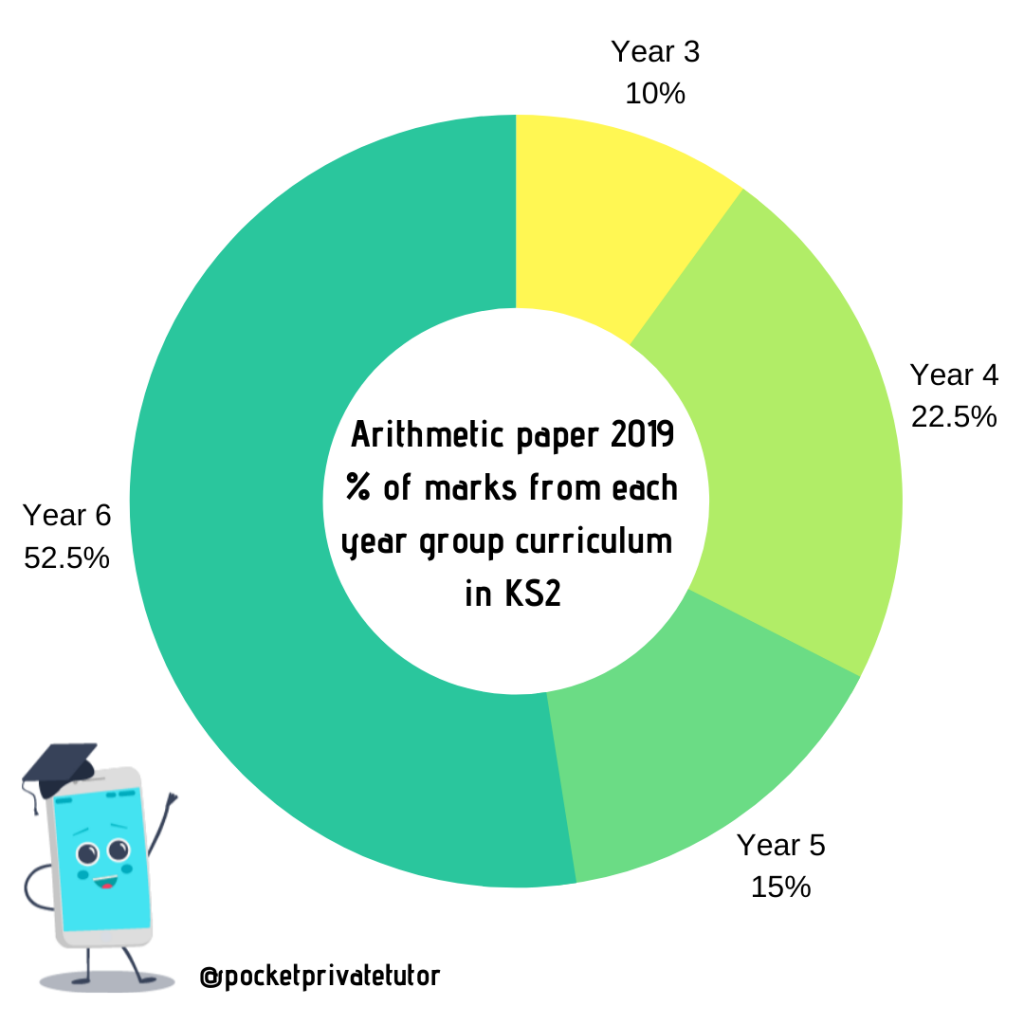Many of the questions in the arithmetic paper come from previous year groups, so it is important to make sure they are secure with their knowledge from previous years too. Spending time filling in any previous gaps is really beneficial. You need to find out exactly which skills your child needs to work on, which may be very different to the other children in their class. If they are missing a skill from a previous year group, there is a chance it may not be covered during their time in Year 6 as there is pressure to move on and cover everything in the Year 6 curriculum in less than 30 weeks!

## Check out my Arithmetic Academy to learn some crucial skills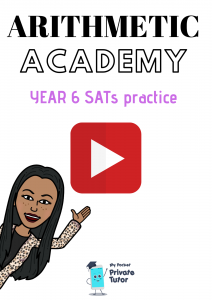This is a 6 part course that covers a range of important areas that commonly appear in Paper 1 and is just £9.99 to download and keep for life.

• Subtraction
• Multiplication
• Division
• Order of operations (using brackets)
• Calculating percentages

Each topic is covered by a video tutorial which runs through five different types of questions for each of the areas, building up in difficulty until we get to a level required in Year 6. All of the 5 question types have and can appear in Paper 1.

Questions are identical in format to what you would find in the Arithmetic Papers so this course make a fantastic revision aid and a way of identifying any gaps that need working on. You can learn everything you need in the video tutorials and the beauty is that you can rewind them to listen or watch again and again.

Just click on the video icon on each worksheet to access the video tutorial.